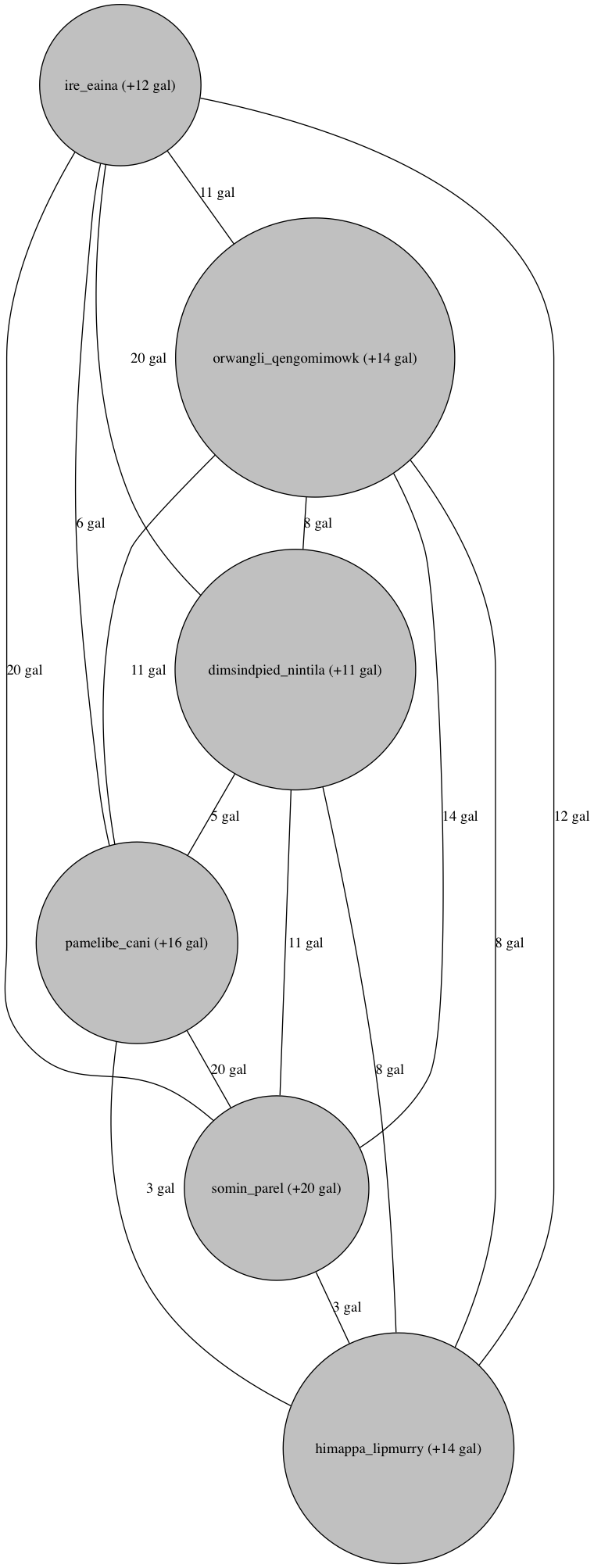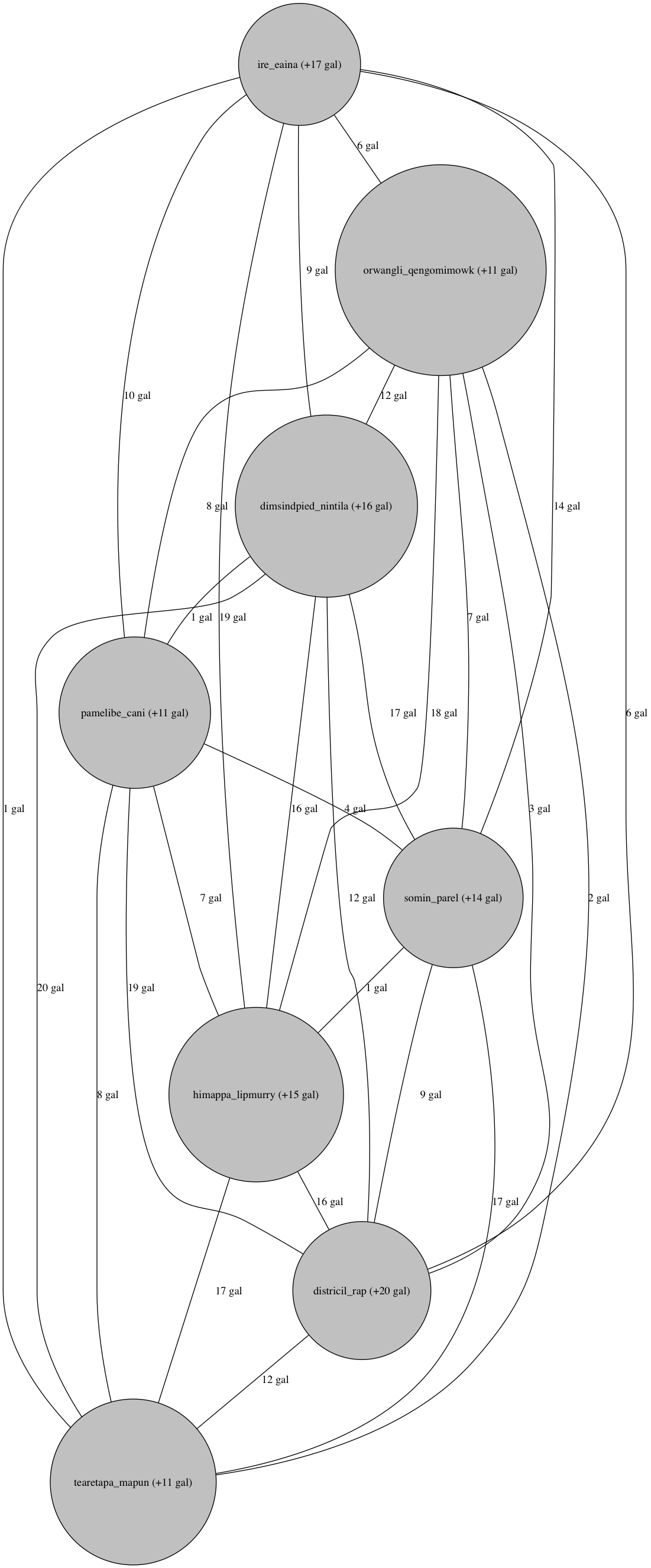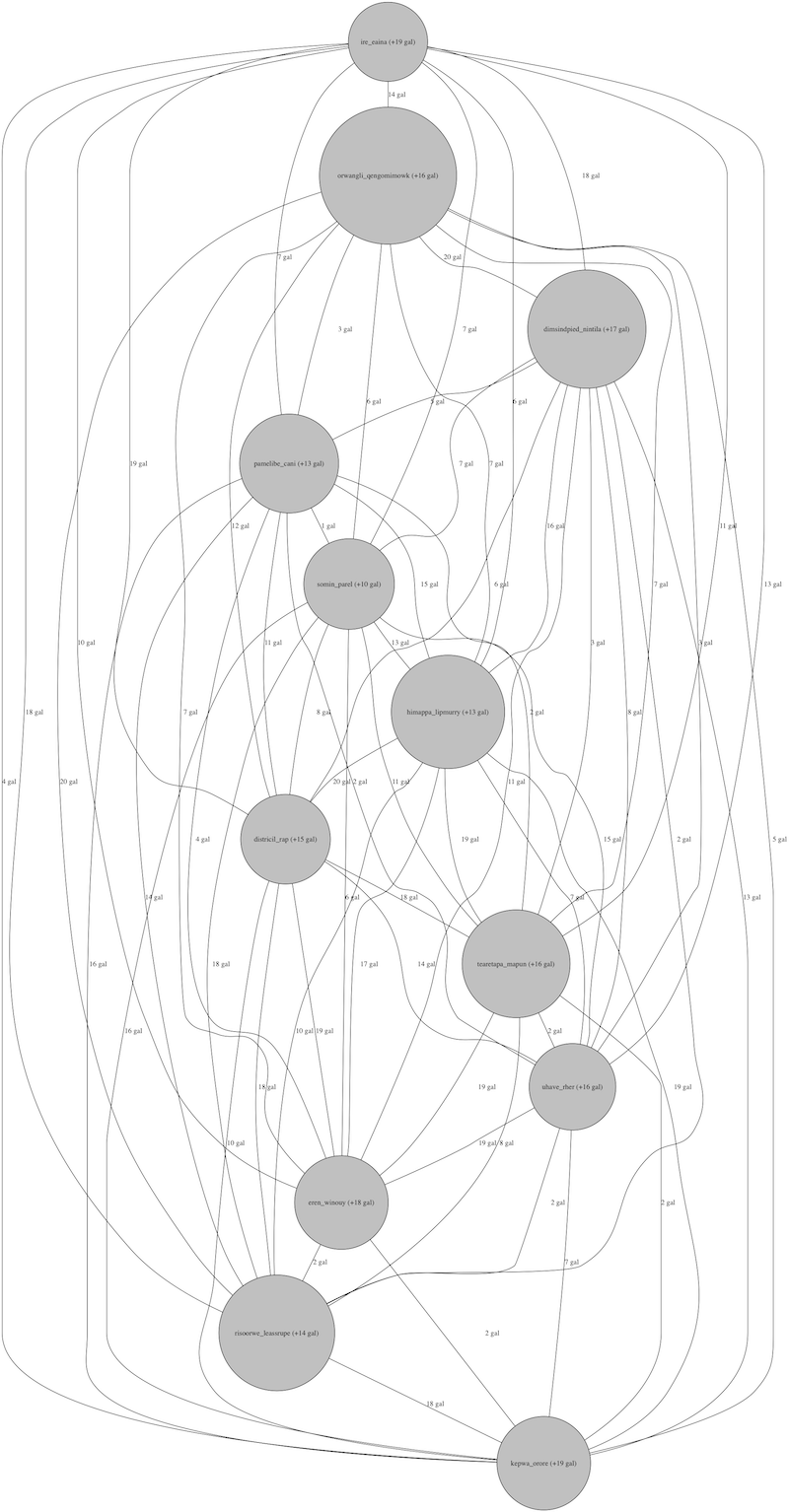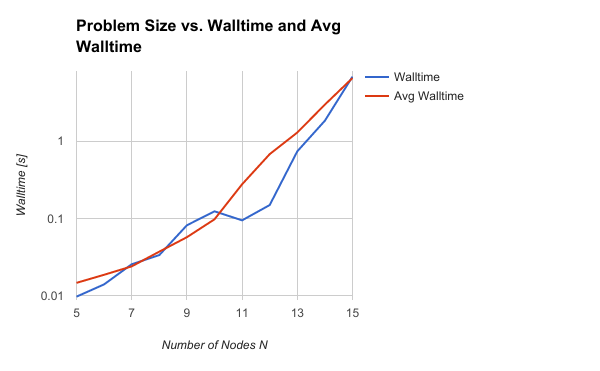# Traveling Schoolteacher Problem

Posted in Java

## The Traveling Schoolteacher Problem

The Traveling Schoolteacher Problem (TSTP) is a variation on the Traveling Salesperson Problem (TSP).

The Traveling Schoolteacher Problem supposes a schoolteacher that is traveling from school to school in order to give lessons at different schools. Being a poor schoolteacher, they are only able to afford an older car that gets bad mileage and has a small gas tank.

After visiting each school, the schoolteacher receives payment from the school, in the currency of $$P$$ gallons of gasoline. Different schools pay the teacher different amounts of gasoline, further complicating matters. The schoolteacher's car has a small gas tank that can only hold a maximum of $$M$$ gallons of gas, and the schoolteacher cannot travel with cans of gasoline. Any gasoline the schoolteacher receives above $$M$$ gallons of gas must be left behind.

Traveling from a source node to a target node incurs a cost of $$C$$ gallons of gas, deducted from the gas tank's total at the source node.

In an attempt to minimize losses and avoid running out of gas, the traveling schoolteacher must plan out a route that both avoids running out of gas and minimizes the total distance traveled.

## The Mathematical Model

To represent this problem in the computer, we can use a graph - just like the Traveling Salesperson Problem solution - but modified a bit. Like the TSP, we can also solve the Traveling Schoolteacher Problem with recursive backtracking.

Each edge will represent a cost in gas, and each school arrived at will result in a payment in gas. Thus, the "real cost" of an edge will change depending on the state of the gas tank and the path taken to arrive there.

We can add a number to each node to represent the amount of gas that that school pays the schoolteacher. We can use a number in each edge to represent the amount of gas that it costs to travel from one school to another. The backtracking solution will explore various paths through teh graph, keeping track of the gas tank's running total and rejecting any paths that lead to an empty gas tank.

## The Pseudocode

Revisiting the original Traveling Salesperson Problem, the recursive backtracking method we implemented was described with the following pseudocode:

explore(neighbors):

if(no more unvisited neighbors):
# This is the base case.
if total distance is less than current minimum:
save path and new minimum

else:
# This is the recursive case.
if current distance is greater than current minimum:
skip
else:
for neighbor in unvisited neighbors:
visit neighbor
explore(new_neighbors)
unvisit neighbor


To modify this to solve the Traveling Schoolteacher Problem, we want to make a few additions:

• Check if the gas tank is empty, and if so, don't explore this path
• Check if the current distance is greater than our current minimum-distance path through the graph
• Add gas from each school to the gas tank (up to the tank's maximum), subtract gas from each path from the gas tank

This results in the following pseudocode:

explore(neighbors):

if(no more unvisited neighbors):
# This is the base case.
if total distance is less than current minimum:
save path and new minimum

else:
# This is the recursive case.
if gas tank is below empty:
skip
if current distance is greater than current minimum:
skip
else:
add gas from this school to gas tank
for neighbor in unvisited neighbors:
visit neighbor
deduct gas to get to neighbor from gas tank

explore(new_neighbors)

unvisit neighbor
add gas back into gas tank


Once you have a backtracking algorithm for the original Traveling Salesperson Problem TSP, it's quite easy to make the few modifications required to solve the Traveling Schoolteacher Problem TSTP.

## The Java Code

The Java code to solve the TSTP is organized into several classes:

• TSTP class - implements the recursive backtracking solution method, and owns temporary variables used by backtracking.
• Node class - the Node is a lightweight class that stores an integer, representing the amount of gas this school pays the teacher. (Note, Node stores no links. Graph links handled by Guava.)
• Edge class - the Edge class is a lightweight class that stores an integer for each edge, representing the amount of gas this path costs to travel.
• RandomNodeGraph - a static class that builds random graphs with nodes and edges pre-populated with values. Parameters like connectivity and maximum gas tank capacity can be passed to introduce variation and ensure the graph is solvable (or not).

### TSTP Class Fields and Methods

The TSTP class implements several fields to store the graph, and to store temporary information about the current solution during recursive backtracking (such that it is accessible by each instance of the recursive method).

The class stores the current route in an integer array, along with the current path distance, the current minimum distance, and the current state of the gas tank.

The TSTP class has a public solve() method, which calls a private recursive backtracking explore() method to solve the problem.

The recursive method will have a base case and a recursive case.

• The base case is that we have visited all cities on the graph. Check if this is a new solution, and if so, save it.

• In the recursive case, we explore all solutions possible starting at the current node (passed in as a parameter), having already made N choices (passed in as a parameter). We do this by making a choice (and marking the node as visited), then exploring the consequences (through a recursive call), then unmaking the choice (rmarking the node as unvisited).

Here is the explore method header:

    /** Recursive backtracking method:
explore possible solutions starting
at this node, having made nchoices */
public void explore(Node node, int nchoices) {


### Explore: Base Case

The base case begins by checking if the minimum distance has been set, and if so, whether the current distance is larger than the minimum distance. If so, this route is abandoned; otherwise, we have a new solution.

        if(nchoices == graphSize) {
//
// BASE CASE
//
if(this.this_distance < this.min_distance || this.min_distance < 0) {
this.min_distance = this.this_distance;
printSolution();
} else {
printFailure();
}

} else {


### Explore: Recursive Case

Next, the recursive case will explore each of the possible choices open to it by iterating over each choice available at a node, and for each node, choosing it, exploring the results, and unchoosing it.

        } else {
//
// RECURSIVE CASE
//
if(this.gas_tank <= 0) {
// Bummer, man.
return;
}
if(this.min_distance > 0 && this.this_distance > this.min_distance) {
// Give up, there's no hope.
return;
}

// Now the teacher teaches,
// Now the teacher gets some gas.
// If the tank is full...  bummer, man.
this.gas_tank += Math.min(this.tankSize, this.gas_tank + node.pay);

// For each neighbor:
for(Node neighbor : neighbors) {
if(neighbor.visited == false) {

int distance_btwn = -10000;

// Using a for loop,
// but there should only be one edge.
for( Edge edge : graph.edgesConnecting(node, neighbor) ) {
distance_btwn = edge.cost;
}

// Make a choice
this.route[nchoices] = neighbor.id;
neighbor.visit();
this.this_distance += distance_btwn;

// Explore the consequences
explore(neighbor,nchoices+1);

// Unmake the choice
this.route[nchoices] = -1;
neighbor.unvisit();
this.this_distance -= distance_btwn;
}
// Move on to the next choice (continue loop)
}
} // End base/recursive case
}


## Example Graphs

Just so you can see what they look like, here are a couple of graphs generated for the traveling schoolteacher problem:## Results

### Walltime vs. Number of Nodes

The following is a plot of walltime versus number of nodes:This plot shows that the traveling schoolteacher problem is solved faster than the traveling salesperson problem. This makes sense - for a given city, many of the possible routes can be eliminated from the list of routes to explore, due to the additional constraint of the gas tank needing to remain full. If there are only 2 gallons of gas in the tank, this constrains the choices of nodes to explore to those requiring 2 gallons of gas or less.

This is particularly true for the scenario used when generating the above graph - the constraint of the gas tank size is used to construct an "interesting" graph tand ensure that we don't end up with a graph where the traveling schoolteacher gets "stuck" somewhere without enough gas to continue. Here is the relevant section of the RandomNodeGraph.java class.

The getNextCost() method generates a random edge between two cities, with a $$\frac{T}{T+1}$$ percent chance of it being impossible for the teacher to travel that route due to a gas tank that's too small:

    private static int getNextCost(int T) {
Random r = new Random();
return 1+r.nextInt(T);
}


Likewise, here is the getNextPay() method, which generates a random amount of pay (in gas) that the

    private static int getNextPay(int T) {
Random r = new Random();
return (int)(0.5*T + 0.5*(r.nextInt(T)+1));
}


Here is a link to the full RandomNodeGraph.java file on the charlesreid1.com git server, contained in the tsp repository (which has several codes related to the traveling salesperson problem): https://git.charlesreid1.com/charlesreid1/tsp/src/master/schoolteacher-guava/RandomNodeGraph.java

And here is a link to the tsp repository on the same charlesreid1.com git server: https://git.charlesreid1.com/charlesreid1/tsp/src/master/schoolteacher-guava

In a city with 10 routes connecting to other cities, having edges that the schoolteacher cannot travel, or nodes that limit the amount of gas the schoolteacher receives, can constrain the number of possible routes and reduce the number of routes that need to be explored. The methods above ensure that this will not happen very often, but that it will happen some of the time. These routes that can be eliminated can lead to a significant reduction in computational time.

## Conclusion

This project shows how easy it is to utilize the Guava library to solve computational problems in Java and create computing benchmarks and graphs of scaling behavior. The original code to solve the traveling salesperson problem using recursive backtracking was fairly straightforward to implement on a graph, and extending this code to implement additional constraints and solve the traveling schoolteacher problem was surprisingly easy to do.

Fitting the scaling behavior to a line and computing the slope would make the scaling study more quantitative, and needs to be done, but unfortunately the Google Sheets tool does not have this capability, so this information will be done with Python (scipy linear algebra package) and comparisons between the TSP and TSTP slopes will be added later.

1. "tsp (git repository)." Charles Reid. Modified 7 April 2017. Accesssed 30 April 2017. <https://git.charlesreid1.com/charlesreid1/tsp>

2. "Solving the Traveling Salesperson Problem with Java and Guava." Charles Reid. 23 March 2017. Accessed 30 April 2017. <https://charlesreid1.github.io/solving-the-traveling-salesperson-problem-with-java-and-guava.html>

3. "Better Timing of Guava Traveling Salesperson Problem Code: Timing Scripts." Charles Reid. 1 April 2017. Accessed 30 April 2017. <https://charlesreid1.github.io/better-timing-of-guava-traveling-salesperson-problem-code-timing-scripts.html>